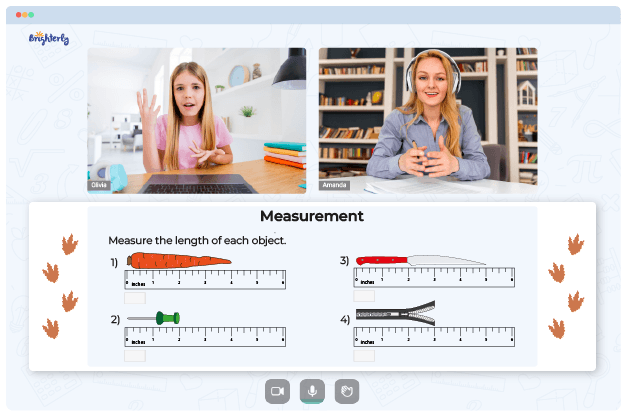# Proportion Word Problems Worksheets

Word problems are essential in math lessons because they show students how math is applied in real life and how to gain the skills they will use for the rest of their life! For this reason, there should be a way to make mastering this skill as fun as possible so that students are engaged enough to learn and understand the concept! A proportion word problem worksheet that shows that two ratios are equal can help.

## Finding Your Way around the Proportion Word Problem Worksheet

With this worksheet, students will solve word problems that involve searching for proportions and finding values ​​when given a ratio. They will compare the two ratios to determine which is higher. Students will fill in the blanks to complete the word-based section.

There’s a surefire way to solve the problems in the worksheets. Create an illustration of the situation: this can be a diagram or picture. Describe the numbers’ relationship through proportions. Don’t forget to read the question part of the problem as some students gloss over it and make mistakes.

Math for Kids

Is Your Child Struggling With Math?
1:1 Online Math TutoringThe section with the proportion word problems worksheet answers explains how to solve proportions from word problems. After completing this worksheet, students will be able to solve word problems that involve balance. Sample exercises are solved, and only then practice problems are presented.

## Why Is the Word Problem Sheet Important?

One of the unique features of the proportion word problem worksheet is that it allows students to be more creative in their problem-solving approach. Downloading and solving proportion word problems worksheet with answers PDF will enable them to make more sense in life and solve exercises effectively.### Proportion Word Problems Worksheets PDF

Proportion Word Problems Worksheet### Proportion Word Problems Worksheets PDF

Proportions Word Problems Worksheet### Proportion Word Problems Worksheets PDF

Solving Proportions Word Problems Worksheets### Proportion Word Problems Worksheets PDF

Proportion Word Problems Worksheet With Answers PDF

Visualizing the answers from solving proportions word problems worksheets can make the problem interesting and engaging. Development of these skills through solving proportions and problem worksheets requires step-by-step guidance and practical explanations from experienced tutors. Worksheets develop their ability to be independent thinkers and problem solvers.

### More Proportions Worksheets

Need help with Measurement?• Does your child struggle to grasp measurement concepts?
• Try studying with an online tutor.

Is your child having difficulties with understanding measurement? An online tutor could provide the necessary guidance.